Algebra and Trigonometry 2e

# Chapter 13

### 13.1Sequences and Their Notations

1.

The first five terms are

2.

The first five terms are

3.

The first six terms are ${ 2,5,54,10,250,15 }. { 2,5,54,10,250,15 }.$

4.

$a n = (−1) n+1 9 n a n = (−1) n+1 9 n$

5.

$a n =− 3 n 4n a n =− 3 n 4n$

6.

$a n = e n−3 a n = e n−3$

7.

8.

9.

The first five terms are

### 13.2Arithmetic Sequences

1.

The sequence is arithmetic. The common difference is $–2. –2.$

2.

The sequence is not arithmetic because $3−1≠6−3. 3−1≠6−3.$

3.

4.

$a 2 = 2 a 2 = 2$

5.

6.

$a n = 53 − 3 n a n = 53 − 3 n$

7.

There are 11 terms in the sequence.

8.

The formula is $T n =10+4n, T n =10+4n,$ and it will take her 42 minutes.

### 13.3Geometric Sequences

1.

The sequence is not geometric because $10 5 ≠ 15 10 10 5 ≠ 15 10$ .

2.

The sequence is geometric. The common ratio is $1 5 1 5$ .

3.

${ 18,6,2, 2 3 , 2 9 } { 18,6,2, 2 3 , 2 9 }$

4.

5.

$a 6 =16,384 a 6 =16,384$

6.

$a n =− (−3) n−1 a n =− (−3) n−1$

7.
1. The number of hits will be about 333.

### 13.4Series and Their Notations

1.

38

2.

$26.4 26.4$

3.

$328 328$

4.

$−280 −280$

5.

$2,025 6. $≈2,000.00 ≈2,000.00$ 7. 9,840 8.$275,513.31

9.

The sum is not defined.

10.

The sum of the infinite series is defined.

11.

The sum of the infinite series is defined.

12.

3

13.

The series is not geometric.

14.

$− 3 11 − 3 11$

15.

$32,775.87 ### 13.5Counting Principles 1. 7 2. There are 60 possible breakfast specials. 3. 120 4. 60 5. 12 6. $P(7,7)=5,040 P(7,7)=5,040$ 7. $P(7,5)=2,520 P(7,5)=2,520$ 8. $C(10,3)=120 C(10,3)=120$ 9. 64 sundaes 10. 840 ### 13.6Binomial Theorem 1. 1. 35 2. 330 2. 1. $x 5 −5 x 4 y+10 x 3 y 2 −10 x 2 y 3 +5x y 4 − y 5 x 5 −5 x 4 y+10 x 3 y 2 −10 x 2 y 3 +5x y 4 − y 5$ 2. $8 x 3 +60 x 2 y+150x y 2 +125 y 3 8 x 3 +60 x 2 y+150x y 2 +125 y 3$ 3. $−10,206 x 4 y 5 −10,206 x 4 y 5$ ### 13.7Probability 1. Outcome Probability Heads $1212$ Tails $1212$ 2. $2 3 2 3$ 3. $7 13 7 13$ 4. $2 13 2 13$ 5. $5 6 5 6$ 6. ### 13.1 Section Exercises 1. A sequence is an ordered list of numbers that can be either finite or infinite in number. When a finite sequence is defined by a formula, its domain is a subset of the non-negative integers. When an infinite sequence is defined by a formula, its domain is all positive or all non-negative integers. 3. Yes, both sets go on indefinitely, so they are both infinite sequences. 5. A factorial is the product of a positive integer and all the positive integers below it. An exclamation point is used to indicate the operation. Answers may vary. An example of the benefit of using factorial notation is when indicating the product It is much easier to write than it is to write out $13⋅12⋅11⋅10⋅9⋅8⋅7⋅6⋅5⋅4⋅3⋅2⋅1. 13⋅12⋅11⋅10⋅9⋅8⋅7⋅6⋅5⋅4⋅3⋅2⋅1.$ 7. First four terms: $−8,− 16 3 ,−4,− 16 5 −8,− 16 3 ,−4,− 16 5$ 9. First four terms: $2, 1 2 , 8 27 , 1 4 2, 1 2 , 8 27 , 1 4$ . 11. First four terms: $1.25,−5,20,−80 1.25,−5,20,−80$ . 13. First four terms: $1 3 , 4 5 , 9 7 , 16 9 1 3 , 4 5 , 9 7 , 16 9$ . 15. First four terms: $− 4 5 ,4,−20,100 − 4 5 ,4,−20,100$ 17. $1 3 , 4 5 , 9 7 , 16 9 , 25 11 ,31,44,59 1 3 , 4 5 , 9 7 , 16 9 , 25 11 ,31,44,59$ 19. $−0.6,−3,−15,−20,−375,−80,−9375,−320 −0.6,−3,−15,−20,−375,−80,−9375,−320$ 21. $a n = n 2 +3 a n = n 2 +3$ 23. 25. $a n = ( − 1 2 ) n−1 a n = ( − 1 2 ) n−1$ 27. First five terms: $3,−9,27,−81,243 3,−9,27,−81,243$ 29. First five terms: $−1,1,−9, 27 11 , 891 5 −1,1,−9, 27 11 , 891 5$ 31. 33. $2,10,12, 14 5 , 4 5 ,2,10,12 2,10,12, 14 5 , 4 5 ,2,10,12$ 35. $a 1 =−8, a n = a n−1 +n a 1 =−8, a n = a n−1 +n$ 37. $a 1 =35, a n = a n−1 +3 a 1 =35, a n = a n−1 +3$ 39. $720 720$ 41. $665,280 665,280$ 43. First four terms: $1, 1 2 , 2 3 , 3 2 1, 1 2 , 2 3 , 3 2$ 45. First four terms: $−1,2, 6 5 , 24 11 −1,2, 6 5 , 24 11$ 47.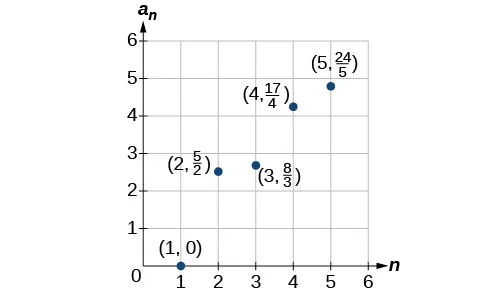49.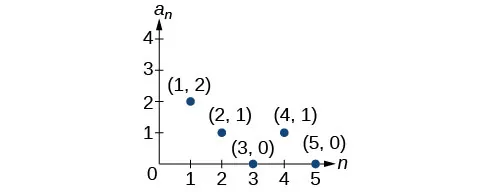51.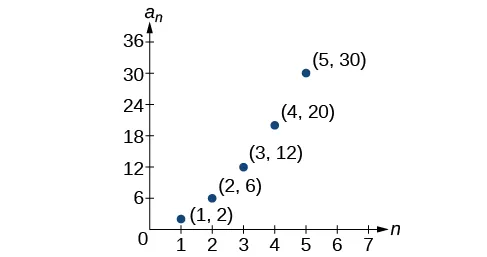53. $a n = 2 n−2 a n = 2 n−2$ 55. $a 1 =6, a n =2 a n−1 −5 a 1 =6, a n =2 a n−1 −5$ 57. First five terms: $29 37 29 37$, $152 111 152 111$, $716 333 716 333$, $3188 999 3188 999$, $13724 2997 13724 2997$ 59. First five terms: 2, 3, 5, 17, 65537 61. $a 10 =7,257,600 a 10 =7,257,600$ 63. First six terms: 0.042, 0.146, 0.875, 2.385, 4.708 65. First four terms: 5.975, 2.765, 185.743, 1057.25, 6023.521 67. If $a n =−421 a n =−421$ is a term in the sequence, then solving the equation $−421=−6−8n −421=−6−8n$ for $n n$ will yield a non-negative integer. However, if $−421=−6−8n, −421=−6−8n,$ then $n=51.875 n=51.875$ so $a n =−421 a n =−421$ is not a term in the sequence. 69. $a 1 =1, a 2 =0, a n = a n−1 − a n−2 a 1 =1, a 2 =0, a n = a n−1 − a n−2$ 71. $(n+2)! (n−1)! = (n+2)·(n+1)·(n)·(n−1)·...·3·2·1 (n−1)·...·3·2·1 =n(n+1)(n+2)= n 3 +3 n 2 +2n (n+2)! (n−1)! = (n+2)·(n+1)·(n)·(n−1)·...·3·2·1 (n−1)·...·3·2·1 =n(n+1)(n+2)= n 3 +3 n 2 +2n$ ### 13.2 Section Exercises 1. A sequence where each successive term of the sequence increases (or decreases) by a constant value. 3. We find whether the difference between all consecutive terms is the same. This is the same as saying that the sequence has a common difference. 5. Both arithmetic sequences and linear functions have a constant rate of change. They are different because their domains are not the same; linear functions are defined for all real numbers, and arithmetic sequences are defined for natural numbers or a subset of the natural numbers. 7. The common difference is $1 2 1 2$ 9. The sequence is not arithmetic because $16−4≠64−16. 16−4≠64−16.$ 11. $0, 2 3 , 4 3 ,2, 8 3 0, 2 3 , 4 3 ,2, 8 3$ 13. $0 , − 5 , − 10 , − 15 , − 20 0 , − 5 , − 10 , − 15 , − 20$ 15. $a 4 =19 a 4 =19$ 17. $a 6 =41 a 6 =41$ 19. $a 1 =2 a 1 =2$ 21. $a 1 =5 a 1 =5$ 23. $a 1 =6 a 1 =6$ 25. $a 21 =−13.5 a 21 =−13.5$ 27. $−19,−20.4,−21.8,−23.2,−24.6 −19,−20.4,−21.8,−23.2,−24.6$ 29. 31. 33. 35. 37. 39. $a 1 =4; a n = a n−1 +7; a 14 =95 a 1 =4; a n = a n−1 +7; a 14 =95$ 41. First five terms: $20,16,12,8,4. 20,16,12,8,4.$ 43. $a n =1+2n a n =1+2n$ 45. $a n =−105+100n a n =−105+100n$ 47. $a n =1.8n a n =1.8n$ 49. $a n =13.1+2.7n a n =13.1+2.7n$ 51. $a n = 1 3 n− 1 3 a n = 1 3 n− 1 3$ 53. There are 10 terms in the sequence. 55. There are 6 terms in the sequence. 57. The graph does not represent an arithmetic sequence. 59.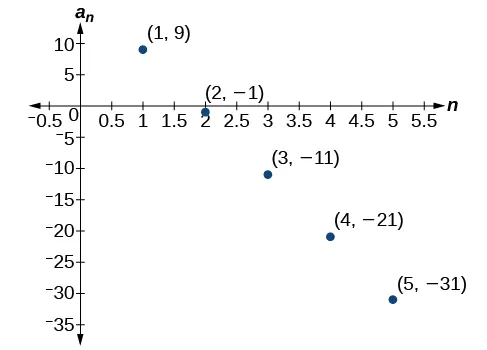61. $1,4,7,10,13,16,19 1,4,7,10,13,16,19$ 63.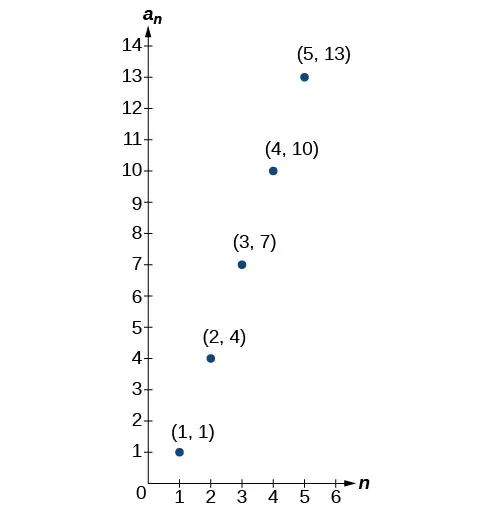65.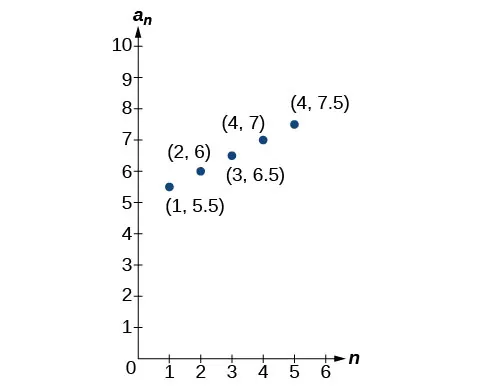67. Answers will vary. Examples: $a n =20.6n a n =20.6n$ and $a n =2+20.4n. a n =2+20.4n.$ 69. $a 11 =−17a+38b a 11 =−17a+38b$ 71. The sequence begins to have negative values at the 13th term, $a 13 =− 1 3 a 13 =− 1 3$ 73. Answers will vary. Check to see that the sequence is arithmetic. Example: Recursive formula: $a 1 =3, a n = a n−1 −3. a 1 =3, a n = a n−1 −3.$ First 4 terms: $3,0,−3,−6 a 31 =−87 3,0,−3,−6 a 31 =−87$ ### 13.3 Section Exercises 1. A sequence in which the ratio between any two consecutive terms is constant. 3. Divide each term in a sequence by the preceding term. If the resulting quotients are equal, then the sequence is geometric. 5. Both geometric sequences and exponential functions have a constant ratio. However, their domains are not the same. Exponential functions are defined for all real numbers, and geometric sequences are defined only for positive integers. Another difference is that the base of a geometric sequence (the common ratio) can be negative, but the base of an exponential function must be positive. 7. The common ratio is $−2 −2$ 9. The sequence is geometric. The common ratio is 2. 11. The sequence is geometric. The common ratio is $− 1 2 . − 1 2 .$ 13. The sequence is geometric. The common ratio is $5. 5.$ 15. $5,1, 1 5 , 1 25 , 1 125 5,1, 1 5 , 1 25 , 1 125$ 17. $800,400,200,100,50 800,400,200,100,50$ 19. $a 4 =− 16 27 a 4 =− 16 27$ 21. $a 7 =− 2 729 a 7 =− 2 729$ 23. $7,1.4,0.28,0.056,0.0112 7,1.4,0.28,0.056,0.0112$ 25. $a = 1 −32, a n = 1 2 a n−1 a = 1 −32, a n = 1 2 a n−1$ 27. $a 1 =10, a n =−0.3 a n−1 a 1 =10, a n =−0.3 a n−1$ 29. $a 1 = 3 5 , a n = 1 6 a n−1 a 1 = 3 5 , a n = 1 6 a n−1$ 31. $a 1 = 1 512 , a n =−4 a n−1 a 1 = 1 512 , a n =−4 a n−1$ 33. $12,−6,3,− 3 2 , 3 4 12,−6,3,− 3 2 , 3 4$ 35. $a n = 3 n−1 a n = 3 n−1$ 37. $a n =0.8⋅ (−5) n−1 a n =0.8⋅ (−5) n−1$ 39. $a n =− ( 4 5 ) n−1 a n =− ( 4 5 ) n−1$ 41. $a n =3⋅ ( − 1 3 ) n−1 a n =3⋅ ( − 1 3 ) n−1$ 43. $a 12 = 1 177,147 a 12 = 1 177,147$ 45. There are $12 12$ terms in the sequence. 47. The graph does not represent a geometric sequence. 49.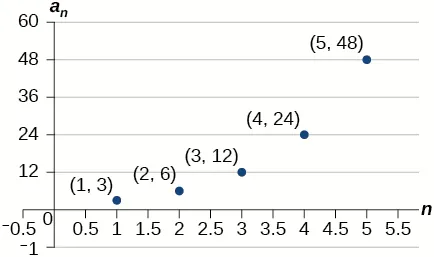51. Answers will vary. Examples: $a 1 =800, a n =0.5a n−1 a 1 =800, a n =0.5a n−1$ and $a 1 =12.5, a n =4a n−1 a 1 =12.5, a n =4a n−1$ 53. $a 5 =256b a 5 =256b$ 55. The sequence exceeds $100 100$ at the 14th term, $a 14 ≈107. a 14 ≈107.$ 57. $a 4 =− 32 3 a 4 =− 32 3$ is the first non-integer value 59. Answers will vary. Example: Explicit formula with a decimal common ratio: $a n =400⋅ 0.5 n−1 ; a n =400⋅ 0.5 n−1 ;$ First 4 terms: $400,200,100,50; a 8 =3.125 400,200,100,50; a 8 =3.125$ ### 13.4 Section Exercises 1. An $nth nth$ partial sum is the sum of the first $n n$ terms of a sequence. 3. A geometric series is the sum of the terms in a geometric sequence. 5. An annuity is a series of regular equal payments that earn a constant compounded interest. 7. $∑ n=0 4 5n ∑ n=0 4 5n$ 9. $∑ k=1 5 4 ∑ k=1 5 4$ 11. $∑ k=1 20 8k+2 ∑ k=1 20 8k+2$ 13. $S 5 = 5( 3 2 + 7 2 ) 2 S 5 = 5( 3 2 + 7 2 ) 2$ 15. $S 13 = 13( 3.2+5.6 ) 2 S 13 = 13( 3.2+5.6 ) 2$ 17. $∑ k=1 7 8⋅ 0.5 k−1 ∑ k=1 7 8⋅ 0.5 k−1$ 19. $S 5 = 9( 1− ( 1 3 ) 5 ) 1− 1 3 = 121 9 ≈13.44 S 5 = 9( 1− ( 1 3 ) 5 ) 1− 1 3 = 121 9 ≈13.44$ 21. $S 11 = 64( 1− 0.2 11 ) 1−0.2 = 781,249,984 9,765,625 ≈80 S 11 = 64( 1− 0.2 11 ) 1−0.2 = 781,249,984 9,765,625 ≈80$ 23. The series is defined. $S= 2 1−0.8 S= 2 1−0.8$ 25. The series is defined. $S= −1 1−( − 1 2 ) S= −1 1−( − 1 2 )$ 27.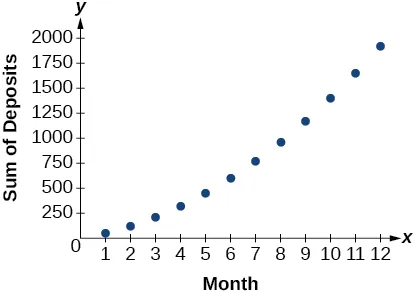29. Sample answer: The graph of $S n S n$ seems to be approaching 1. This makes sense because $∑ k=1 ∞ ( 1 2 ) k ∑ k=1 ∞ ( 1 2 ) k$ is a defined infinite geometric series with $S= 1 2 1–( 1 2 ) =1. S= 1 2 1–( 1 2 ) =1.$ 31. 49 33. 254 35. $S 7 = 147 2 S 7 = 147 2$ 37. $S 11 = 55 2 S 11 = 55 2$ 39. $S 7 =5208.4 S 7 =5208.4$ 41. $S 10 =− 1023 256 S 10 =− 1023 256$ 43. $S=− 4 3 S=− 4 3$ 45. $S=9.2 S=9.2$ 47.$3,705.42

49.

$695,823.97 51. $a k =30−k a k =30−k$ 53. 9 terms 55. $r= 4 5 r= 4 5$ 57.$400 per month

59.

420 feet

61.

12 feet

### 13.5 Section Exercises

1.

There are $m+n m+n$ ways for either event $A A$ or event $B B$ to occur.

3.

The addition principle is applied when determining the total possible of outcomes of either event occurring. The multiplication principle is applied when determining the total possible outcomes of both events occurring. The word “or” usually implies an addition problem. The word “and” usually implies a multiplication problem.

5.

A combination; $C(n,r)= n! (n−r)!r! C(n,r)= n! (n−r)!r!$

7.

$4+2=6 4+2=6$

9.

$5+4+7=16 5+4+7=16$

11.

$2×6=12 2×6=12$

13.

$10 3 =1000 10 3 =1000$

15.

$P(5,2)=20 P(5,2)=20$

17.

$P(3,3)=6 P(3,3)=6$

19.

$P(11,5)=55,440 P(11,5)=55,440$

21.

$C(12,4)=495 C(12,4)=495$

23.

$C(7,6)=7 C(7,6)=7$

25.

$2 10 =1024 2 10 =1024$

27.

$2 12 =4096 2 12 =4096$

29.

$2 9 =512 2 9 =512$

31.

$8! 3! =6720 8! 3! =6720$

33.

$12! 3!2!3!4! 12! 3!2!3!4!$

35.

9

37.

Yes, for the trivial cases $r=0 r=0$ and $r=1. r=1.$ If $r=0, r=0,$ then $C(n,r)=P(n,r)=1. C(n,r)=P(n,r)=1.$ If $r=1, r=1,$ then $r=1, r=1,$ $C(n,r)=P(n,r)=n. C(n,r)=P(n,r)=n.$

39.

$6! 2! ×4!=8640 6! 2! ×4!=8640$

41.

$6−3+8−3=8 6−3+8−3=8$

43.

$4×2×5=40 4×2×5=40$

45.

$4×12×3=144 4×12×3=144$

47.

$P(15,9)=1,816,214,400 P(15,9)=1,816,214,400$

49.

$C(10,3)×C(6,5)×C(5,2)=7,200 C(10,3)×C(6,5)×C(5,2)=7,200$

51.

$2 11 =2048 2 11 =2048$

53.

$20! 6!6!8! =116,396,280 20! 6!6!8! =116,396,280$

### 13.6 Section Exercises

1.

A binomial coefficient is an alternative way of denoting the combination $C(n,r). C(n,r).$ It is defined as $( n r )=C(n,r)= n! r!(n−r)! . ( n r )=C(n,r)= n! r!(n−r)! .$

3.

The Binomial Theorem is defined as $(x+y) n = ∑ k=0 n ( n k ) x n−k y k (x+y) n = ∑ k=0 n ( n k ) x n−k y k$ and can be used to expand any binomial.

5.

15

7.

35

9.

10

11.

12,376

13.

$64 a 3 −48 a 2 b+12a b 2 − b 3 64 a 3 −48 a 2 b+12a b 2 − b 3$

15.

$27 a 3 +54 a 2 b+36a b 2 +8 b 3 27 a 3 +54 a 2 b+36a b 2 +8 b 3$

17.

$1024 x 5 +2560 x 4 y+2560 x 3 y 2 +1280 x 2 y 3 +320x y 4 +32 y 5 1024 x 5 +2560 x 4 y+2560 x 3 y 2 +1280 x 2 y 3 +320x y 4 +32 y 5$

19.

$1024 x 5 −3840 x 4 y+5760 x 3 y 2 −4320 x 2 y 3 +1620x y 4 −243 y 5 1024 x 5 −3840 x 4 y+5760 x 3 y 2 −4320 x 2 y 3 +1620x y 4 −243 y 5$

21.

$1 x 4 + 8 x 3 y + 24 x 2 y 2 + 32 x y 3 + 16 y 4 1 x 4 + 8 x 3 y + 24 x 2 y 2 + 32 x y 3 + 16 y 4$

23.

$a 17 +17 a 16 b+136 a 15 b 2 a 17 +17 a 16 b+136 a 15 b 2$

25.

$a 15 −30 a 14 b+420 a 13 b 2 a 15 −30 a 14 b+420 a 13 b 2$

27.

$3,486,784,401 a 20 +23,245,229,340 a 19 b+73,609,892,910 a 18 b 2 3,486,784,401 a 20 +23,245,229,340 a 19 b+73,609,892,910 a 18 b 2$

29.

$x 24 −8 x 21 y +28 x 18 y x 24 −8 x 21 y +28 x 18 y$

31.

$−720 x 2 y 3 −720 x 2 y 3$

33.

$220,812,466,875,000 y 7 220,812,466,875,000 y 7$

35.

$35 x 3 y 4 35 x 3 y 4$

37.

$1,082,565 a 3 b 16 1,082,565 a 3 b 16$

39.

$1152 y 2 x 7 1152 y 2 x 7$

41.

$f 2 (x)= x 4 +12 x 3 f 2 (x)= x 4 +12 x 3$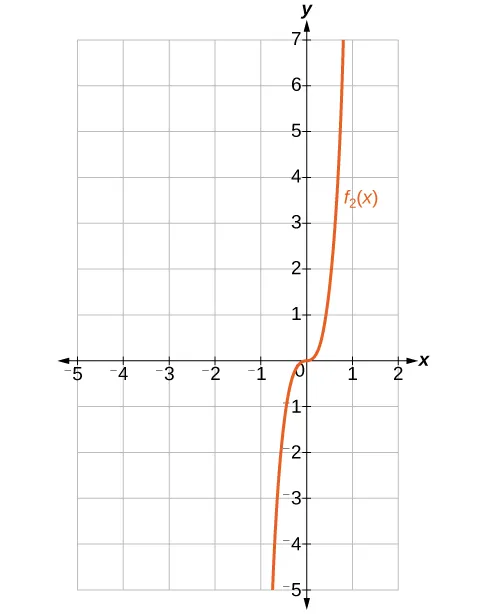43.

$f 4 (x)= x 4 +12 x 3 +54 x 2 +108x f 4 (x)= x 4 +12 x 3 +54 x 2 +108x$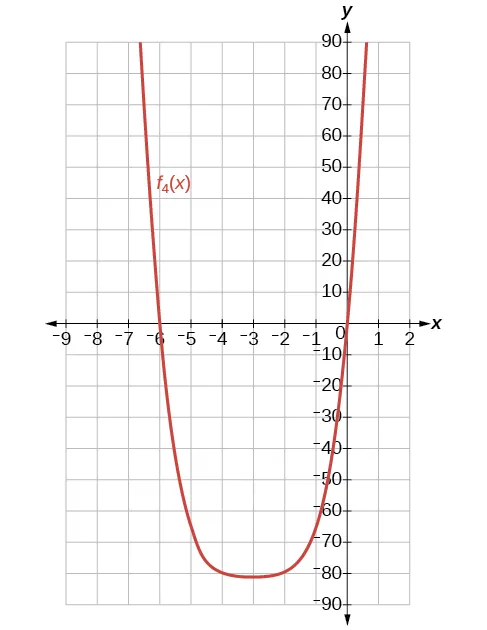45.

$590,625 x 5 y 2 590,625 x 5 y 2$

47.

$k−1 k−1$

49.

The expression $( x 3 +2 y 2 −z) 5 ( x 3 +2 y 2 −z) 5$ cannot be expanded using the Binomial Theorem because it cannot be rewritten as a binomial.

### 13.7 Section Exercises

1.

probability; The probability of an event is restricted to values between $0 0$ and $1, 1,$ inclusive of $0 0$ and $1. 1.$

3.

An experiment is an activity with an observable result.

5.

The probability of the union of two events occurring is a number that describes the likelihood that at least one of the events from a probability model occurs. In both a union of sets and a union of events the union includes either or both. The difference is that a union of sets results in another set, while the union of events is a probability, so it is always a numerical value between $0 0$ and $1. 1.$

7.

$1 2 . 1 2 .$

9.

$5 8 . 5 8 .$

11.

$1 2 . 1 2 .$

13.

$3 8 . 3 8 .$

15.

$1 4 . 1 4 .$

17.

$3 4 . 3 4 .$

19.

$3 8 . 3 8 .$

21.

$1 8 . 1 8 .$

23.

$15 16 . 15 16 .$

25.

$5 8 . 5 8 .$

27.

$1 13 . 1 13 .$

29.

$1 26 . 1 26 .$

31.

$12 13 . 12 13 .$

33.
 1 2 3 4 5 6 1 (1,1)2 (1,2)3 (1,3)4 (1,4)5 (1,5)6 (1,6)7 2 (2,1)3 (2,2)4 (2,3)5 (2,4)6 (2,5)7 (2,6)8 3 (3,1)4 (3,2)5 (3,3)6 (3,4)7 (3,5)8 (3,6)9 4 (4,1)5 (4,2)6 (4,3)7 (4,4)8 (4,5)9 (4,6)10 5 (5,1)6 (5,2)7 (5,3)8 (5,4)9 (5,5)10 (5,6)11 6 (6,1)7 (6,2)8 (6,3)9 (6,4)10 (6,5)11 (6,6)12
35.

$5 12 . 5 12 .$

37.

$0. 0.$

39.

$4 9 . 4 9 .$

41.

$1 4 . 1 4 .$

43.

$5 8 5 8$

45.

$8 13 8 13$

47.

$C(12,5) C(48,5) = 1 2162 C(12,5) C(48,5) = 1 2162$

49.

$C(12,3)C(36,2) C(48,5) = 175 2162 C(12,3)C(36,2) C(48,5) = 175 2162$

51.

$C(20,3)C(60,17) C(80,20) ≈12.49% C(20,3)C(60,17) C(80,20) ≈12.49%$

53.

$C(20,5)C(60,15) C(80,20) ≈23.33% C(20,5)C(60,15) C(80,20) ≈23.33%$

55.

$20.50+23.33−12.49=31.34% 20.50+23.33−12.49=31.34%$

57.

$C(40000000,1)C(277000000,4) C(317000000,5) =36.78% C(40000000,1)C(277000000,4) C(317000000,5) =36.78%$

59.

$C(40000000,4)C(277000000,1) C(317000000,5) =0.11% C(40000000,4)C(277000000,1) C(317000000,5) =0.11%$

### Review Exercises

1.

$2,4,7,11 2,4,7,11$

3.

$13,103,1003,10003 13,103,1003,10003$

5.

The sequence is arithmetic. The common difference is $d= 5 3 . d= 5 3 .$

7.

$18,10,2,−6,−14 18,10,2,−6,−14$

9.

$a 1 =−20, a n = a n−1 +10 a 1 =−20, a n = a n−1 +10$

11.

$a n = 1 3 n+ 13 24 a n = 1 3 n+ 13 24$

13.

$r=2 r=2$

15.

4, 16, 64, 256, 1024

17.

$3,12,48,192,768 3,12,48,192,768$

19.

$a n =− 1 5 ⋅ ( 1 3 ) n−1 a n =− 1 5 ⋅ ( 1 3 ) n−1$

21.

$∑ m=0 5 ( 1 2 m+5 ). ∑ m=0 5 ( 1 2 m+5 ).$

23.

$S 11 =110 S 11 =110$

25.

$S 9 ≈23.95 S 9 ≈23.95$

27.

$S= 135 4 S= 135 4$

29.

\$5,617.61

31.

6

33.

$10 4 =10,000 10 4 =10,000$

35.

$P(18,4)=73,440 P(18,4)=73,440$

37.

$C( 15,6 )=5005 C( 15,6 )=5005$

39.

$2 50 =1.13× 10 15 2 50 =1.13× 10 15$

41.

$8! 3!2! =3360 8! 3!2! =3360$

43.

$490,314 490,314$

45.

$131,072 a 17 +1,114,112 a 16 b+4,456,448 a 15 b 2 131,072 a 17 +1,114,112 a 16 b+4,456,448 a 15 b 2$

47.
 1 2 3 4 5 6 1 1,1 1,2 1,3 1,4 1,5 1,6 2 2,1 2,2 2,3 2,4 2,5 2,6 3 3,1 3,2 3,3 3,4 3,5 3,6 4 4,1 4,2 4,3 4,4 4,5 4,6 5 5,1 5,2 5,3 5,4 5,5 5,6 6 6,1 6,2 6,3 6,4 6,5 6,6
49.

$1 6 1 6$

51.

$5 9 5 9$

53.

$4 9 4 9$

55.

$1− C( 350,8 ) C( 500,8 ) ≈94.4% 1− C( 350,8 ) C( 500,8 ) ≈94.4%$

57.

$C( 150,3 )C( 350,5 ) C( 500,8 ) ≈25.6% C( 150,3 )C( 350,5 ) C( 500,8 ) ≈25.6%$

### Practice Test

1.

$−14,−6,−2,0 −14,−6,−2,0$

3.

The sequence is arithmetic. The common difference is $d=0.9. d=0.9.$

5.

$a 1 =−2, a n = a n−1 − 3 2 ; a 22 =− 67 2 a 1 =−2, a n = a n−1 − 3 2 ; a 22 =− 67 2$

7.

The sequence is geometric. The common ratio is $r= 1 2 . r= 1 2 .$

9.

$a 1 =1, a n =− 1 2 ⋅ a n −1 a 1 =1, a n =− 1 2 ⋅ a n −1$

11.

$∑ k=−3 15 ( 3 k 2 − 5 6 k ) ∑ k=−3 15 ( 3 k 2 − 5 6 k )$

13.

$S 7 =−2604.2 S 7 =−2604.2$

15.

Total in account: $140,355.75; 140,355.75;$ Interest earned: $14,355.75 14,355.75$

17.

$5×3×2×3×2=180 5×3×2×3×2=180$

19.

$C( 15,3 )=455 C( 15,3 )=455$

21.

$10! 2!3!2! =151,200 10! 2!3!2! =151,200$

23.

$429 x 14 16 429 x 14 16$

25.

$4 7 4 7$

27.

$5 7 5 7$

29.

$C( 14,3 )C( 26,4 ) C( 40,7 ) ≈29.2% C( 14,3 )C( 26,4 ) C( 40,7 ) ≈29.2%$

Order a print copy

As an Amazon Associate we earn from qualifying purchases.